# TimeMoney WorldTime, Money and Growth

INVESTMENTSHOMEBORROWINGSAVING

# Capital Growth

### The Effect of Different Rates of Growth (Interest)

Here you can see how 1,000.00 (any currency) grows over a period of 5 years at the yearly interest rate of 10.0% but at different compounding periods, if the fraction of the yearly interest is added to the capital at the end of each each compounding period, as it would be in an interest bearing bank deposit account.

The computations and graphs below are equally valid for a case where 1,000.00 is invested in a business yielding an annual profit as a percentage of the amount invested, and the fraction of the yearly profit is re-invested at the end of the each compounding period.

The compounding periods considered are: yearly (annually), half-yearly (semi-annually), quarterly, monthly, weekly, daily, and continuous.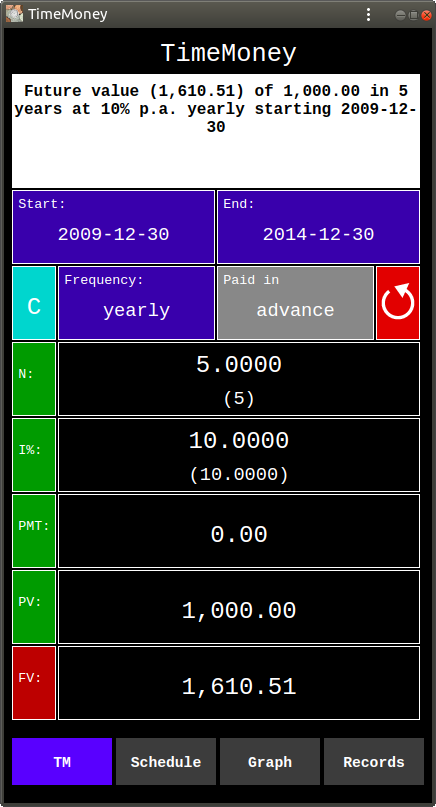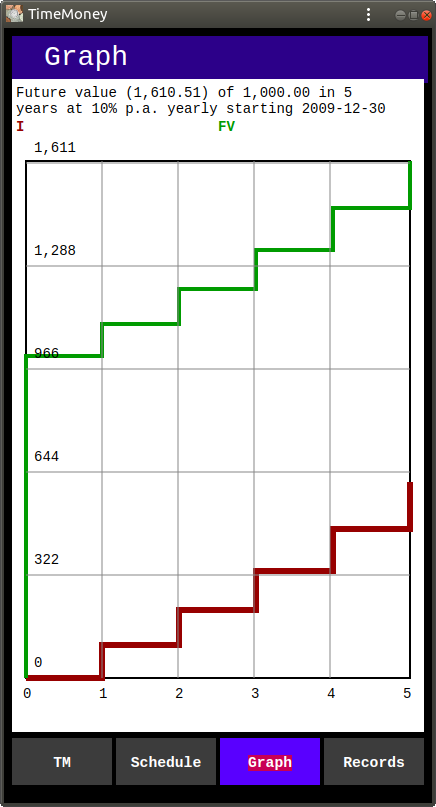This is yearly (annual) compounding. Here the yearly interest is added at the end of each year, and there are 5 compounding periods. And there are 5 steps in each graph line.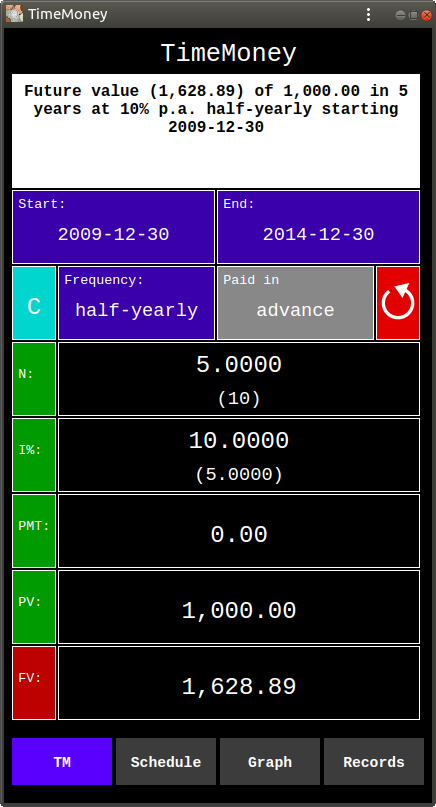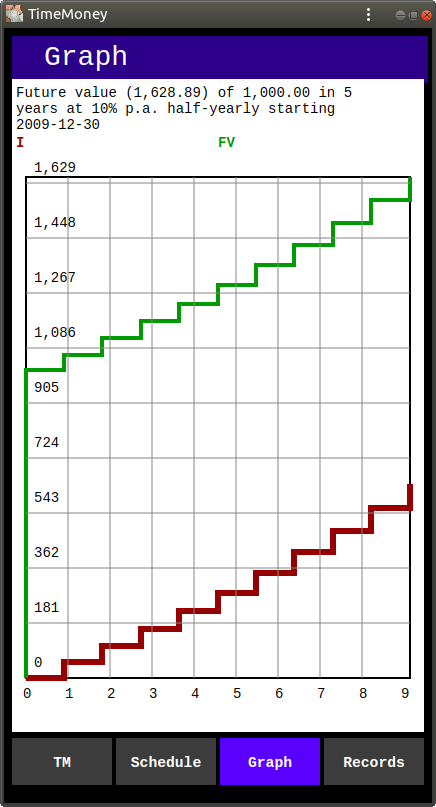This is half-yearly (semi-annual) compounding. Here half of the yearly interest is added at the end of every 6th month, and there are 10 compounding periods. And there are 10 steps in each graph line.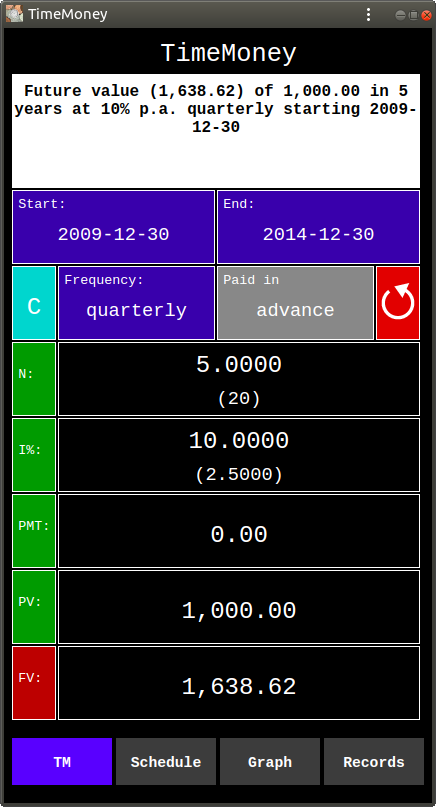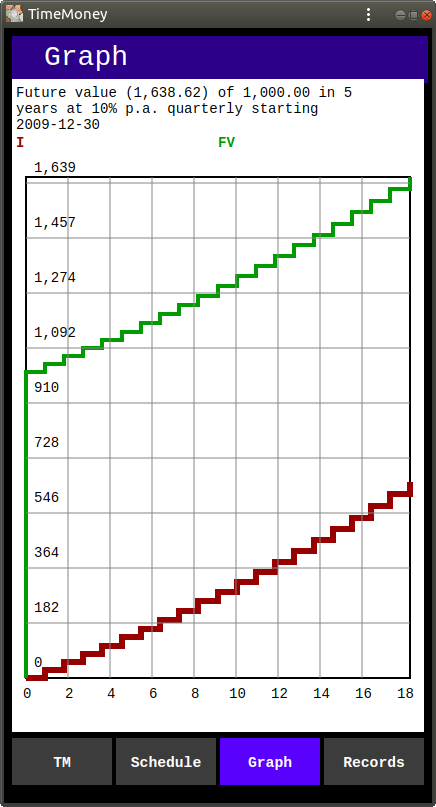This is quarterly compounding. Here one quarter of the yearly interest is added at the end of every 3rd month, and there are 20 compounding periods. And there are 20 steps in each graph line.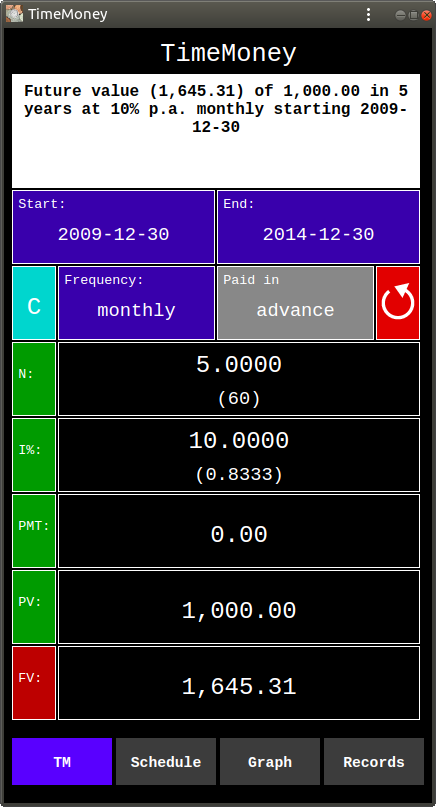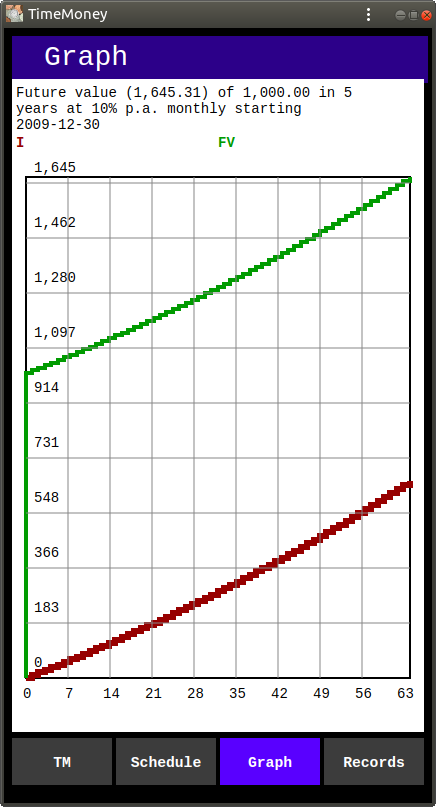This is monthly compounding. Here one twelfth of the yearly interest is added at the end of every month, and there are 60 compounding periods. And there are 60 steps in each graph line.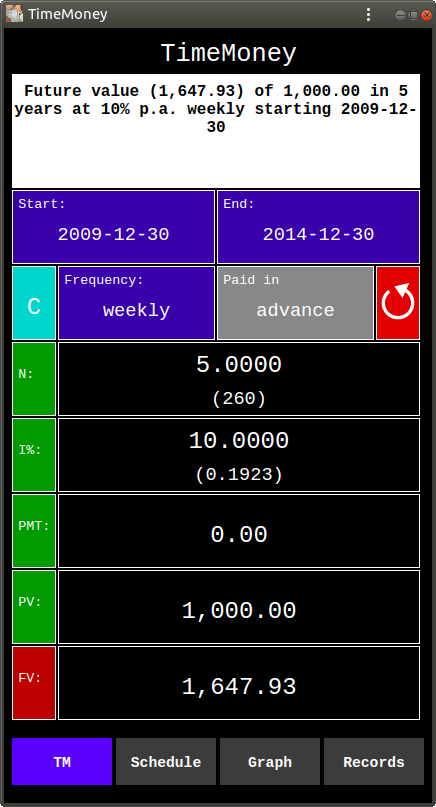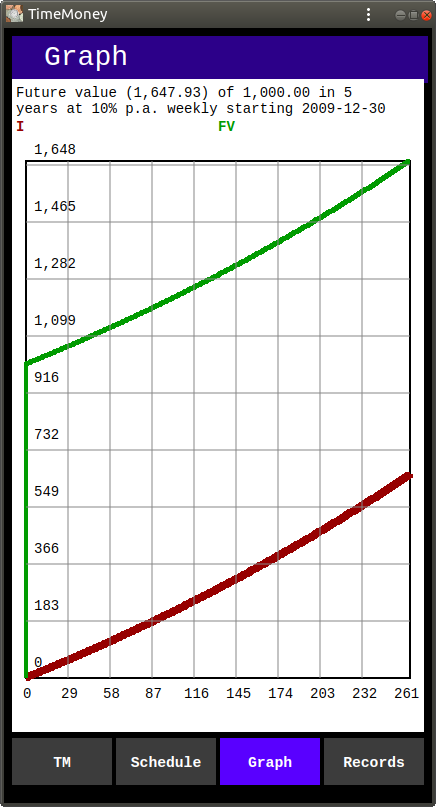This is weekly compounding. Here 1 / 52 of the yearly interest is added at the end of every week, and there are 260 compounding periods. And there are 260 steps in each graph line.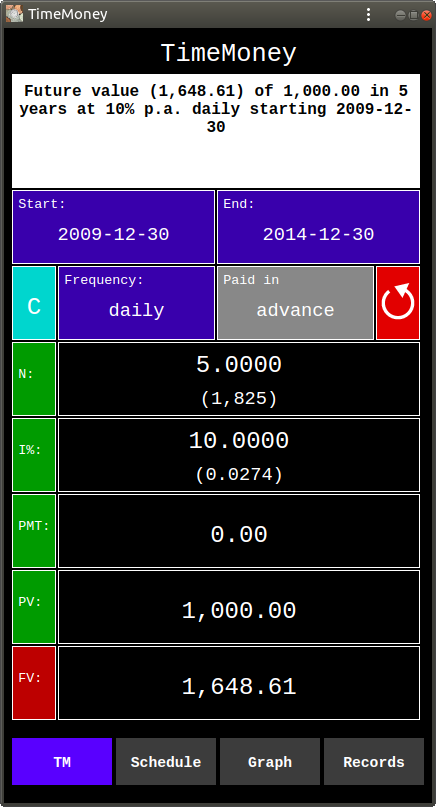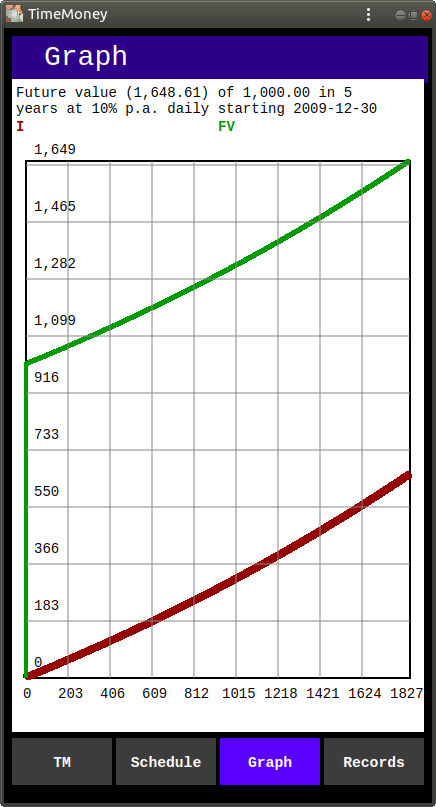This is daily compounding. Here 1 / 365 of the yearly interest is added at the end of every day, and there are 1825 compounding periods. And there are 1825 steps in each graph line.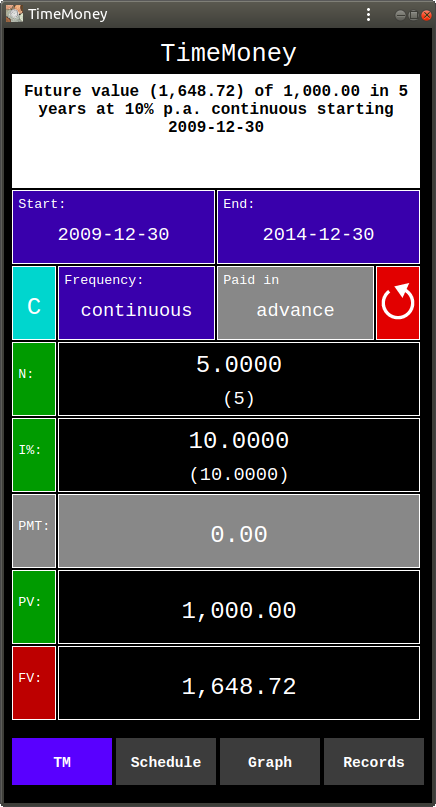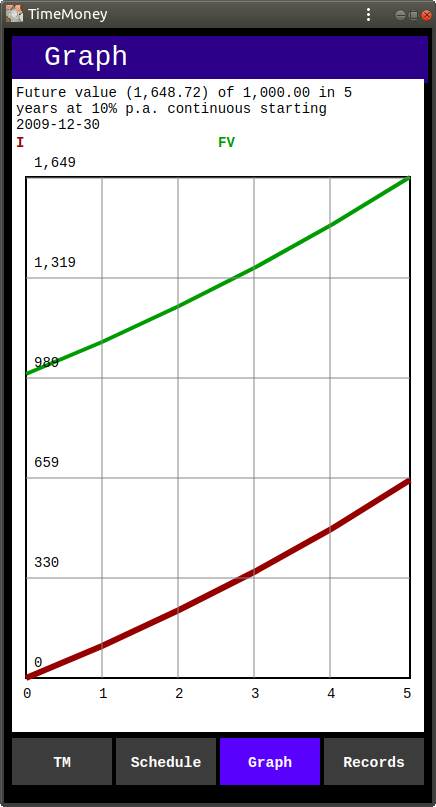This is continuous compounding. Here the yearly interest is added continuously by infinite increments, and there are an infinite number of compounding periods. And each graph line is a smooth curve (without steps).

As we see from above, increasing the number of compounding periods increases the total amount of accumulated interest. But this increase has a limit. And the limit is continuous compounding.

The formula for Future Value (FV) is:

FV = PV * (1 + r / k) n * k

Where:

PV is Present Value,
r is the yearly interest, as a fraction (i / 100)
n is number of years
k is number of compounding periods in a year.

And as k is approaching infinity, the formula becomes:

PV * e r * n

Where: e is the exponential constant (2.71828)

The above computations, schedules, and graphs were performed using TimeMoney by Dalasoft Ltd.

TimeMoney app is a universal Progressive Web Application (PWA) which works on any device (phone, tablet, desktop) on any operating system from your browser, as long as it supports modern JavaScript. And, if you install it on your device, you will be able to start it by clicking its badge on your Home Screen, like any other app on your device.
TimeMoney app is FREE. It has no ads and does not collect any data.
To try it out, use this link: TimeMoney .